/

### Estimating operational risk capital requirements assuming data follows a bi-uniform or a triangular distribution (using maximum likelihood)

Suppose a risk manager believes that an appropriate model for a particular type of operational risk exposure involves the loss,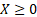, never exceeding an upper limit,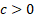, and the probability density function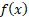taking the form of a bi-uniform distribution: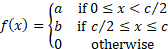whereare all constant.

Suppose we want to estimate maximum likelihood estimators for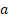,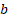andgiven losses of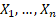, say and hence to estimate a Value-at-Risk for a given confidence level for this loss type, assuming that the probability distribution has the form set out above.

We note that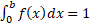for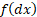to correspond to a probability density function, so: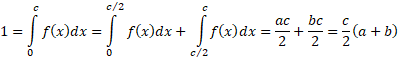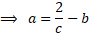Suppose the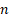losses,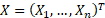, are assumed to be independent draws from a distribution with probability density functionand suppose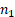of these losses are less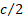and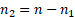are greater than. The likelihood is then: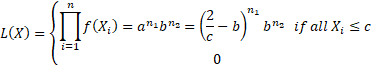This will be maximised for some value that has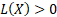, i.e. hasat least as large as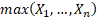. In such circumstances the likelihood is maximised when the log likelihood is maximised which will be when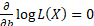, i.e. when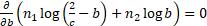, i.e. when: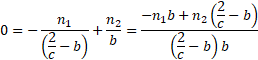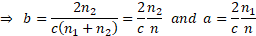(assuming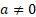and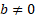)

For these values ofandthe log likelihood is then: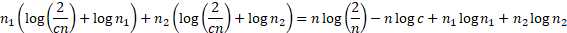In most circumstances this will be maximised whenis as small as possible, providedis still at least as large asso the maximum likelihood estimators are: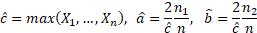However, it is occasionally necessary to consider the case where we select a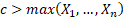and haveand/orequal to zero.

To estimate a VaR at a confidence level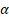we need to find the value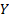for which the loss is expected to exceedonly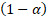% of the time, i.e.such that (if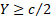):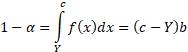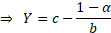A similar approach can be used for tri-uniform distributions or other more complex piecewise uniform distributions.

Somewhat more complex is iftakes the form of a triangular distribution, i.e. where: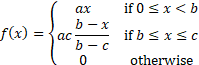where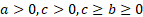are all constant.

Nguyen and McLachlan (2016), when developing a new algorithm for maximum likelihood estimation of triangular or more general polygonal distributions, note that in the case where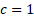and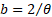then Oliver (1972), “A maximum likelihood oddity”, American Statistician 26, 43–44, indicates that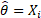for some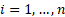and moreover that if the sample is ordered so that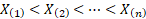then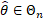where: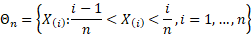Asymptotically, on average, the number of observations in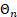appears to be approximately two.

We can apply this reasoning to the more general triangular distribution where we do not knowby again noting that in many cases the log-likelihood will be minimised by settingto be as small as possible, providedis still at least as large as, and then separately handling cases where the likelihood can be improved by selecting a higher value for.